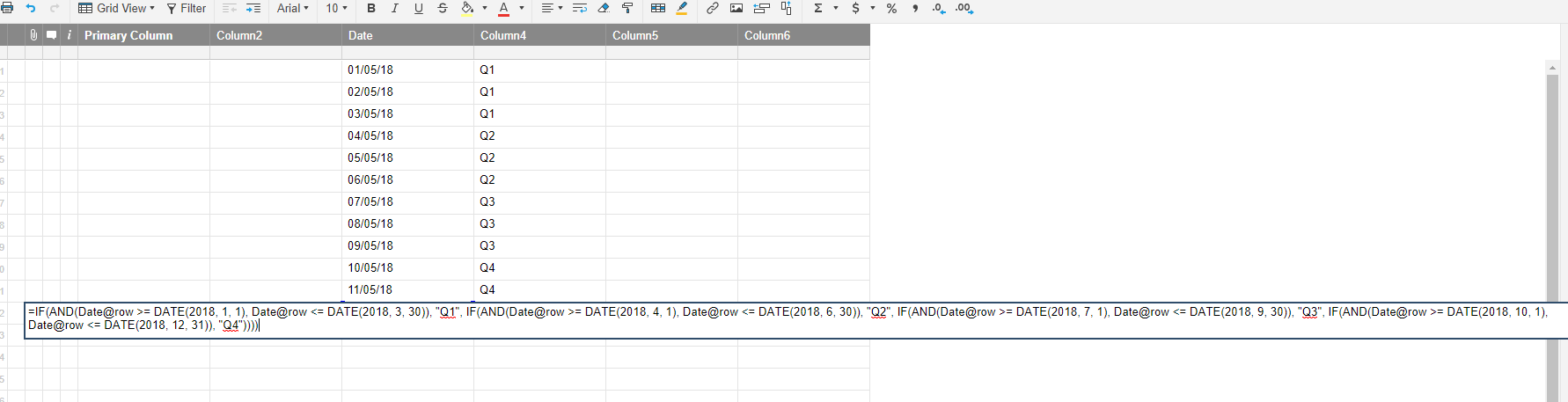# IF formula combined with dates

Hello,

I need help. I am wondering how can I create a formula using the IF function with a range of dates. For example:

If the date is between 01/01/2018 and 03/30/2018, then write 1st Quarter., and if the date is between 04/01/2018 and 06/30/2018, then 2nd. Quarter, etc....

Tags:

• Give this a try (a few minor changes may be needed, explained below)

=IF(AND(Date@row >= DATE(2018, 1, 1), Date@row <= DATE(2018, 3, 30)), "Q1", IF(AND(Date@row >= DATE(2018, 4, 1), Date@row <= DATE(2018, 6, 30)), "Q2", IF(AND(Date@row >= DATE(2018, 7, 1), Date@row <= DATE(2018, 9, 30)), "Q3", IF(AND(Date@row >= DATE(2018, 10, 1), Date@row <= DATE(2018, 12, 31)), "Q4"))))

Where it says Date@row, you only need to change the Date portion to your column name.

"Q1", etc... can be changed to "1st Quarter", etc... or whatever you would like it to say.

The dates that denote the beginning and end of each quarter can be changed to whatever you would like. The format for Smartsheet is DATE(YYYY, MM, DD)• This formula does the same as Paul's example:

="Q" + INT((MONTH(Date@row) + 2) / 3)

without the year.

If it needs to say "1st Quarter" and not "Q1", then you'll need an nested if like Paul's (with the shorter formula to determine 1-4 here) or a LOOKUP() function to return "1st" when search for "1"

The problem with building formulas that look for a specific time frame is that they will need to be changed / maintained as time moves forward.

On January 1st, all dates will have a blank Quarter number.

I'd prefer

=IFERROR("Q" + INT((MONTH(Date@row) + 2) / 3) + "Y" + RIGHT(YEAR(Date@row),2),"not a date")

to return Q1Y18 for 1st quarter this year.

My \$0.02

Craig

• Diggin' it...I always forget about the INT function.

## Help Article Resources

Want to practice working with formulas directly in Smartsheet?

Check out the Formula Handbook template!